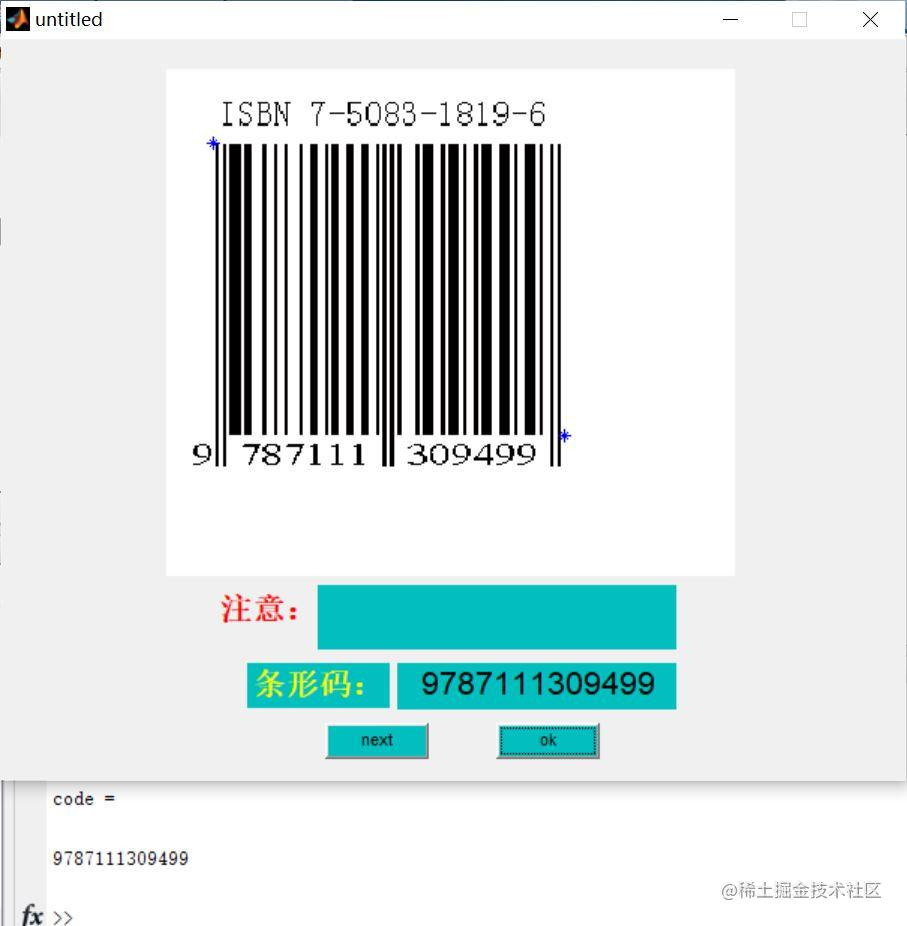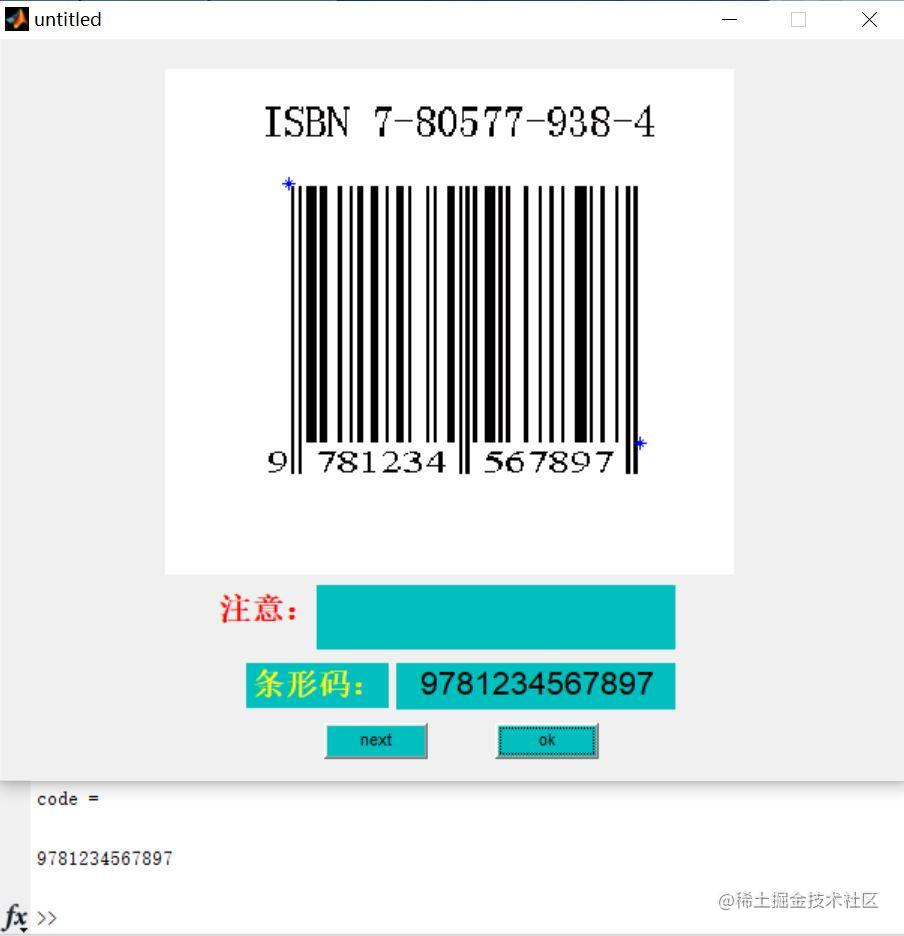# 【条形码识别】基于matlab GUI二维条形码识别【含Matlab源码 607期】

## 二、源代码

``````function varargout = untitled(varargin)
% UNTITLED M-file for untitled.fig
%      UNTITLED, by itself, creates a new UNTITLED or raises the existing
%      singleton*.
%
%      H = UNTITLED returns the handle to a new UNTITLED or the handle to
%      the existing singleton*.
%
%      UNTITLED('CALLBACK',hObject,eventData,handles,...) calls the local
%      function named CALLBACK in UNTITLED.M with the given input arguments.
%
%      UNTITLED('Property','Value',...) creates a new UNTITLED or raises the
%      existing singleton*.  Starting from the left, property value pairs are
%      applied to the GUI before untitled_OpeningFcn gets called.  An
%      unrecognized property name or invalid value makes property application
%      stop.  All inputs are passed to untitled_OpeningFcn via varargin.
%
%      *See GUI Options on GUIDE's Tools menu.  Choose "GUI allows only one
%      instance to run (singleton)".
%

% Edit the above text to modify the response to help untitled

% Begin initialization code - DO NOT EDIT
gui_Singleton = 1;
gui_State = struct('gui_Name',       mfilename, ...
'gui_Singleton',  gui_Singleton, ...
'gui_OpeningFcn', @untitled_OpeningFcn, ...
'gui_OutputFcn',  @untitled_OutputFcn, ...
'gui_LayoutFcn',  [] , ...
'gui_Callback',   []);
if nargin && ischar(varargin{1})
gui_State.gui_Callback = str2func(varargin{1});
end

if nargout
[varargout{1:nargout}] = gui_mainfcn(gui_State, varargin{:});
else
gui_mainfcn(gui_State, varargin{:});
end
% End initialization code - DO NOT EDIT

% --- Executes just before untitled is made visible.
function untitled_OpeningFcn(hObject, eventdata, handles, varargin)
% This function has no output args, see OutputFcn.
% hObject    handle to figure
% eventdata  reserved - to be defined in a future version of MATLAB
% handles    structure with handles and user data (see GUIDATA)
% varargin   command line arguments to untitled (see VARARGIN)

% Choose default command line output for untitled
handles.output = hObject;
axes(handles.axes1);
imshow(image1);

% Update handles structure
guidata(hObject, handles);

% UIWAIT makes untitled wait for user response (see UIRESUME)
% uiwait(handles.figure1);

% --- Outputs from this function are returned to the command line.
function varargout = untitled_OutputFcn(hObject, eventdata, handles)
% varargout  cell array for returning output args (see VARARGOUT);
% hObject    handle to figure
% eventdata  reserved - to be defined in a future version of MATLAB
% handles    structure with handles and user data (see GUIDATA)

% Get default command line output from handles structure
varargout{1} = handles.output;

% --- Executes on mouse press over axes background.

% --- Executes on button press in pushbutton1.
function pushbutton1_Callback(hObject, eventdata, handles)
% hObject    handle to pushbutton1 (see GCBO)
% eventdata  reserved - to be defined in a future version of MATLAB
% handles    structure with handles and user data (see GUIDATA)

global im;
%提取条形码区域构成的矩形的左上角坐标
%%%%%%%%%%%%%%%%%%%%%%%%%%%%%%%%%%%%%%%%%%%%%%%%%%%%%%%%%%%%%%%%%%%%%%%%%%
image=im;
l1=rgb2gray(image);
level=graythresh(l1);
l2=im2bw(l1,level);
l3=~l2;
l4=bwareaopen(l3,50);
l5=~l4;
l6=edge(l1,'canny');
l7=imclose(l6,strel('rectangle',[2,19]));
l8=imopen(l7,strel('rectangle',[2,19]));
l9=imopen(l8,strel('rectangle',[2,19]));
[L,num]=bwlabel(l9,8);
STATS=regionprops(L,'all');
a=length(STATS);
%figure,imshow(L);
%hold on;
b=0;
for i=1:a
temp=STATS(i).BoundingBox;
s=temp(3)*temp(4);
if s>b
b=s;
k=i;%记录面积最大的标记区域的索引值
end
end
%rectangle('position',STATS(k).BoundingBox,'edgecolor','r');%绘制最大标记区域的外接矩形
temp=STATS(k).BoundingBox;
Rx=round(temp(1));Ry=round(temp(2));%提取条形码区域左上角的坐标
Rwidth=round(temp(3));Rlength=round(temp(4));

%初始化
%%%%%%%%%%%%%%%%%%%%%%%%%%%%%%%%%%%%%%%%%%%%%%%%%%%%%%%%%%%%%%%%%%%%%%%%%%
check_left = [13,25,19,61,35,49,47,59,55,11;...	%左边数据编码，奇
39,51,27,33,29,57, 5,17, 9,23];	%左边数据编码，偶
check_right = [114,102,108,66,92,78,80,68,72,116];	%右边数据编码
first_num = [31,20,18,17,12,6,3,10,9,5];    %第一位数据编码
bar = im;  %读输入条形码图片
bar_Gray = rgb2gray(bar);   %将RGB图片转换灰度图

[a_hist x] = imhist(bar_Gray);   %绘制灰度直方图，返回直方图数据向量a_hist，和相应的色彩值向量x

%寻找进行二值化处理的阈值，存放在T中
%%%%%%%%%%%%%%%%%%%%%%%%%%%%%%%%%%%%%%%%%%%%%%%%%%%%%%%%%%%%%%%%%%%

hist_max = [];
if a_hist(1)>a_hist(2)
hist_max = [hist_max 1];
end
x = max(x);
for i=2:x
if a_hist(i)>a_hist(i-1) && a_hist(i)>a_hist(i+1)
hist_max = [hist_max i];
end
end
if a_hist(x)<a_hist(x+1)
hist_max = [hist_max x+1];
end
[m,n] = size(hist_max);
k = 0;
max_1 = 0;
max_2 = 0;
for i=1:n
if k<a_hist(hist_max(i))
k = a_hist(hist_max(i));
max_1 = hist_max(i);
end
end
temp = a_hist(max_1);
a_hist(max_1) = 0;
k = 0;
for i=1:n
if k<a_hist(hist_max(i))
k = a_hist(hist_max(i));
max_2 = hist_max(i);
end
end
a_hist(max_1) = temp;
if max_1>max_2
k = max_1;
max_1 = max_2;
max_2 = k;
end
T = max_1;
k = a_hist(max_1);
for i=max_1:max_2
if k>a_hist(i)
k = a_hist(i);
T = i;
end
end

%%%%%%%%%%%%%%%%%%%%%%%%%%%%%%%%%%%%%%%%%%%%%%%%%%%%%%%%%%%%%%%%%%%%%%%%%

[m,n] = size(bar_Gray); %求灰度图的大小
for i=1:m        %对图像进行二值化处理
for j=1:n
if bar_Gray(i,j)>T    %选择适当的阈值进行二值化处理
bar_10(i,j) = 1;
else
bar_10(i,j) = 0;
end
end
end

## 三、运行结果